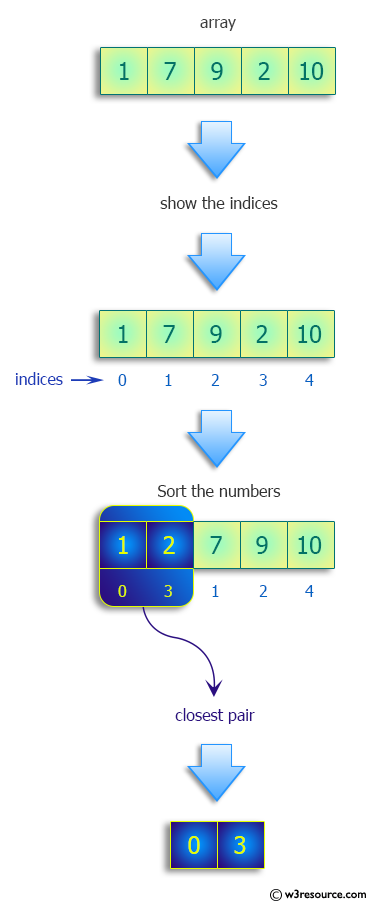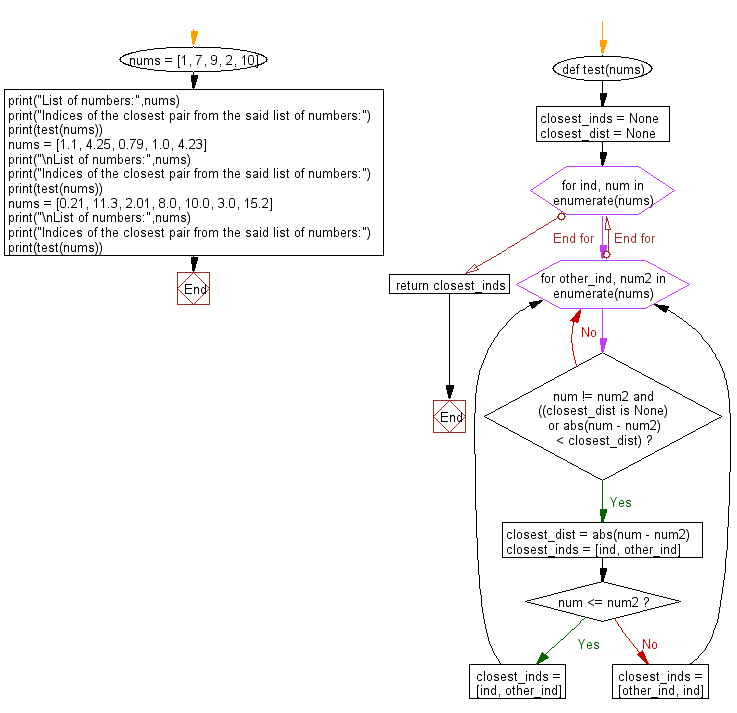﻿ Python: Find the indices of the closest pair from given a list of numbers - w3resource# Python: Find the indices of the closest pair from given a list of numbers

## Python Programming Puzzles: Exercise-66 with Solution

Write a Python program to find the indices of the closest pair from a list of numbers.

```Input: [1, 7, 9, 2, 10]
Output:
[0, 3]

Input: [1.1, 4.25, 0.79, 1.0, 4.23]
Output:
[4, 1]

Input: [0.21, 11.3, 2.01, 8.0, 10.0, 3.0, 15.2]
Output:
[2, 5]
```

Pictorial Presentation:Sample Solution:

Python Code:

``````#License: https://bit.ly/3oLErEI

def test(nums):
closest_inds = None
closest_dist = None
for ind, num in enumerate(nums):
for other_ind, num2 in enumerate(nums):
if num != num2 and ((closest_dist is None) or abs(num - num2) < closest_dist):
closest_dist = abs(num - num2)
closest_inds = [ind, other_ind]
if num <= num2:
closest_inds = [ind, other_ind]
else:
closest_inds = [other_ind, ind]
return closest_inds

nums = [1, 7, 9, 2, 10]
print("List of numbers:",nums)
print("Indices of the closest pair from the said list of numbers:")
print(test(nums))

nums = [1.1, 4.25, 0.79, 1.0, 4.23]
print("\nList of numbers:",nums)
print("Indices of the closest pair from the said list of numbers:")
print(test(nums))
nums = [0.21, 11.3, 2.01, 8.0, 10.0, 3.0, 15.2]
print("\nList of numbers:",nums)
print("Indices of the closest pair from the said list of numbers:")
print(test(nums))
``````

Sample Output:

```List of numbers: [1, 7, 9, 2, 10]
Indices of the closest pair from the said list of numbers:
[0, 3]

List of numbers: [1.1, 4.25, 0.79, 1.0, 4.23]
Indices of the closest pair from the said list of numbers:
[4, 1]

List of numbers: [0.21, 11.3, 2.01, 8.0, 10.0, 3.0, 15.2]
Indices of the closest pair from the said list of numbers:
[2, 5]
```

Flowchart:## Visualize Python code execution:

The following tool visualize what the computer is doing step-by-step as it executes the said program:

Python Code Editor :

Have another way to solve this solution? Contribute your code (and comments) through Disqus.

What is the difficulty level of this exercise?

Test your Programming skills with w3resource's quiz.

﻿

## Python: Tips of the Day

Clamps num within the inclusive range specified by the boundary values x and y:

Example:

```def tips_clamp_num(num,x,y):
return max(min(num, max(x, y)), min(x, y))
print(tips_clamp_num(2, 4, 6))
print(tips_clamp_num(1, -1, -6))
```

Output:

```4
-1
```